# Riemannian metric

Jump to: navigation, search

The metric of a space given by a positive-definite quadratic form. If a local coordinate system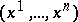is introduced for the space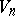and if at each point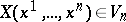functions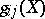,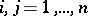,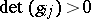,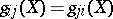, are defined which are the components of a covariant symmetric tensor of the second valency, then this tensor is called the fundamental metric tensor of. The length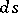of the covariant vectoris expressed using the fundamental tensor: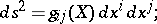the form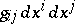is a positive-definite quadratic form. The metric ofdetermined using the form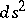is called Riemannian, and a space with a given Riemannian metric introduced into it is called a Riemannian space. The specification of a Riemannian metric on a differentiable manifold means the specification of a Euclidean structure on the tangent spaces of this manifold depending on the points in a differentiable way.

A Riemannian metric is a generalization of the first fundamental form of a surface in three-dimensional Euclidean space — of the internal metric of the surface. The geometry of the spacebased on a definite Riemannian metric is called a Riemannian geometry.

There are generalizations of the concept of a Riemannian metric. Thus, a pseudo-Riemannian metric is defined with the aid of a non-definite non-degenerate quadratic form (see Pseudo-Riemannian space and Relativity theory). A degenerate Riemannian metric, that is, a metric form defined with the aid of functionsfor which, defines a semi-Riemannian space.